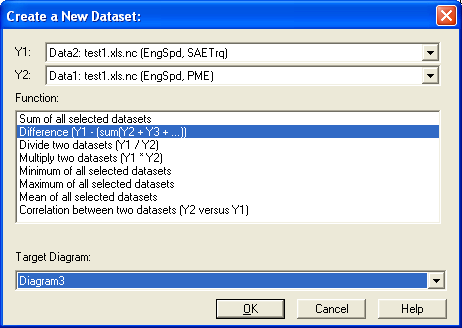Data=>Linear Interpolation¶This function can be used for 2D datasets which have monoton increasing or decreasing x-coordinates.

Mark the first dataset. Press the SHIFT key and right click the second dataset. From the context menu choose the command “Datasets=>2D Interpol Sum/Diff…”.

A new dataset will be created as following:

• The overlapping x-range of the selected datasets will be calculated.
• Inside the overlapping range a new vector with x-coordinates will be calculated, which contains the x-coordinates (xs) of all selected datasets.
• For each dataset the linear interpolated y-coordinates are calculated for the xs-coordinates.
• Then the selected function can be applied (Add, Difference, Multiplication, Divide, Min,Max, Mean, Correlation) (ys).
• The new dataset can then be created (xs, ys).
Version Description
5.14.8 Data can have monoton decreasing x-coordinates.

id-1079412